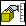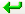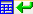> > > To measure properties of parts and assemblies

To measure properties of parts and assemblies
Calculate the properties of parts and assemblies in two different ways: calculate in a global coordinate system or calculate in a reference coordinate system. Inertia can be calculated only in reference coordinates. Principal axis and centroid can be calculated in global and reference coordinates.
1. Click Analysis and then, in the Measure group, clickPart. The Measure Output and Part & Assy Props dialog box opens.
2. If the part or assembly you want is different from the default, select a new part or assembly:
Identify the part. The word defined appears in the field.
Click Part & Assy and select a part using the browser.
3. If you want a specific tolerance, click the Percent check box and enter a value.
4. Select the property to calculate:
In the Calculate (global) area:
1. To calculate the volume, click Volume.
2. To calculate the mass, click Mass.
3. To calculate the density, click Density.
4. To calculate the area, click Area.
5. To calculate the centroid, click Centroid.
6. To calculate the principal axis, click Principal Axes.
7. To record the calculations, click Report.
In the Calculate (Ref Coord) area:
1. To select the reference coordinate, click Ref Coord.
2. To calculate the centroid, click Centroid.
3. To calculate the Inertia, click Inertia.
4. To calculate the principal axis, click Principal Axes.
5. To record the calculations, click Report.
5. To measure the properties of other parts, click Part Assy and select the part.
6. Click the green arrow buttonto send the measurement to a command.
7. Click the green arrow and calculator buttonto send the measurement to the calculator.
The Information section in both Part Assy and Face dialog boxes displays the following:
Max % Error: The percentage error in the calculation.
Units: The current properties units (for example, mm2 for area).
Expand: Additional details are available where appropriate. Click the Expand check box to display the absolute and percentage error in the calculation.